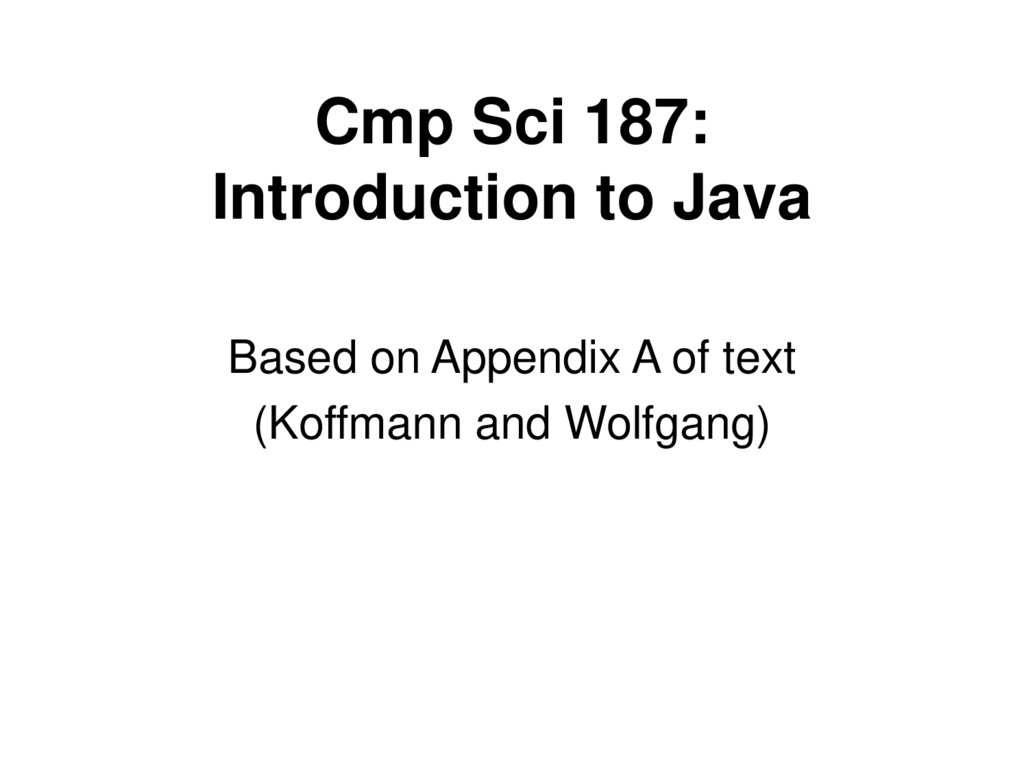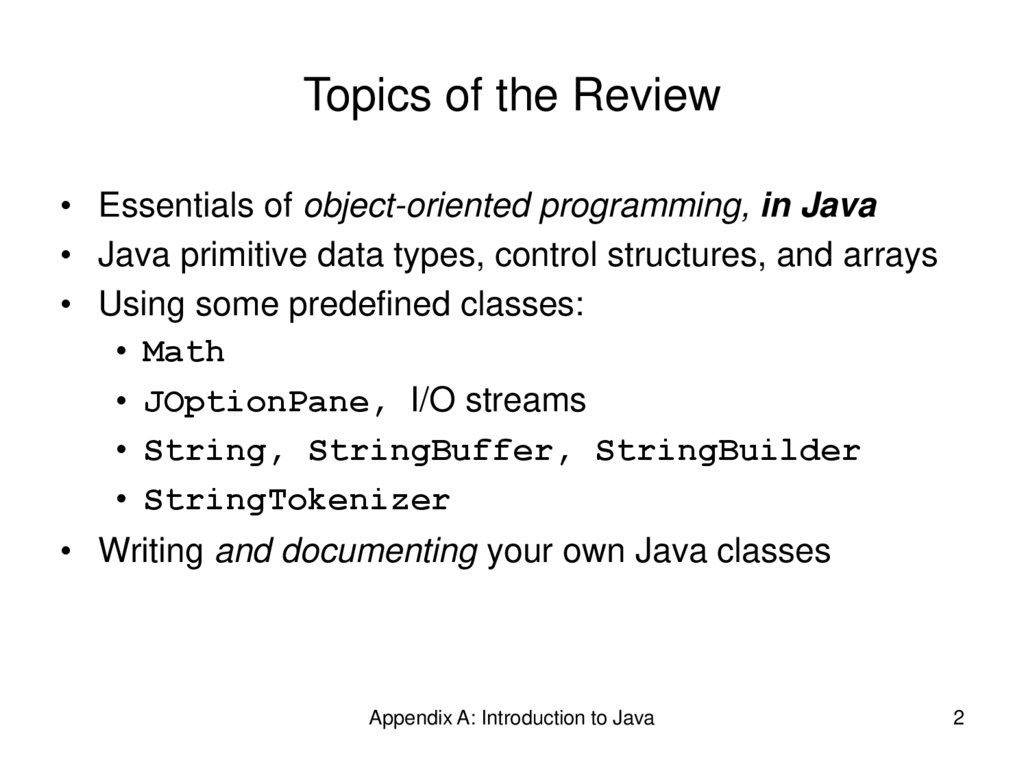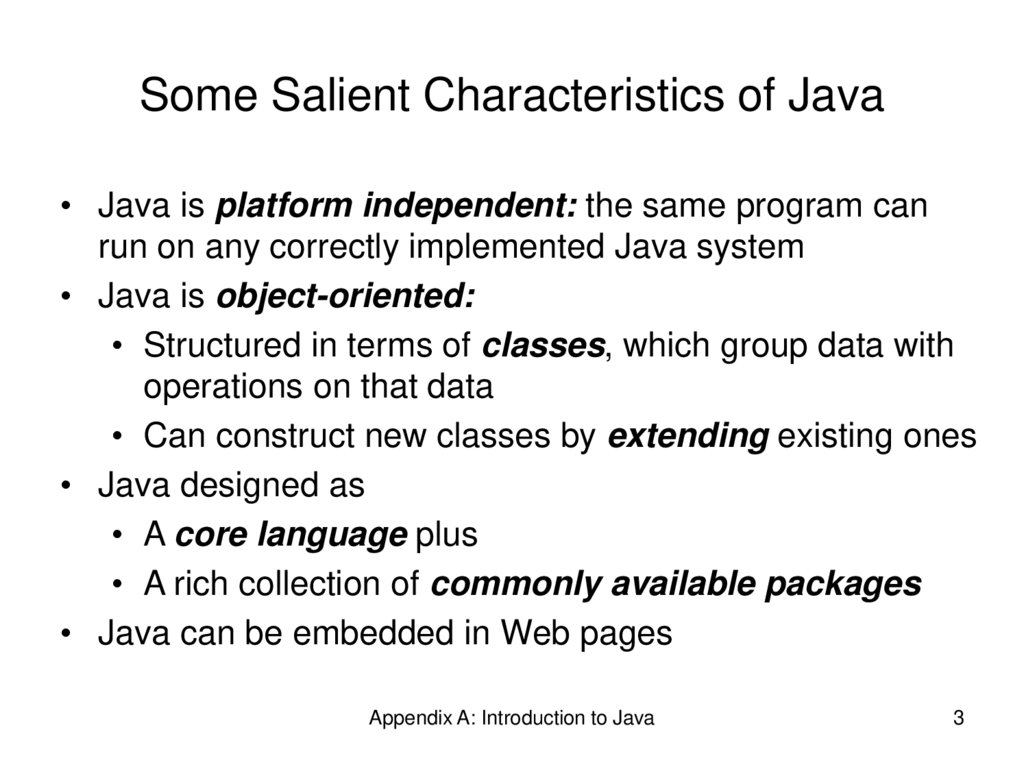# Introduction to Java

## 1. Cmp Sci 187: Introduction to Java

Based on Appendix A of text
(Koffmann and Wolfgang)

## 2. Topics of the Review

• Essentials of object-oriented programming, in Java
• Java primitive data types, control structures, and arrays
• Using some predefined classes:
• Math
• JOptionPane, I/O streams
• String, StringBuffer, StringBuilder
• StringTokenizer
• Writing and documenting your own Java classes
Appendix A: Introduction to Java
2

## 3. Some Salient Characteristics of Java

• Java is platform independent: the same program can
run on any correctly implemented Java system
• Java is object-oriented:
• Structured in terms of classes, which group data with
operations on that data
• Can construct new classes by extending existing ones
• Java designed as
• A core language plus
• A rich collection of commonly available packages
• Java can be embedded in Web pages
Appendix A: Introduction to Java
3

## 4. Java Processing and Execution

• Begin with Java source code in text files: Model.java
• A Java source code compiler produces Java byte code
• Outputs one file per class: Model.class
• May be standalone or part of an IDE
• A Java Virtual Machine loads and executes class files
• May compile them to native code (e.g., x86) internally
Appendix A: Introduction to Java
4

## 5. Compiling and Executing a Java Program

Appendix A: Introduction to Java
5

## 6. Classes and Objects

• The class is the unit of programming
• A Java program is a collection of classes
• Each class definition (usually) in its own .java file
• The file name must match the class name
• A class describes objects (instances)
• Describes their common characteristics: is a blueprint
• Thus all the instances have these same characteristics
• These characteristics are:
• Data fields for each object
• Methods (operations) that do work on the objects
Appendix A: Introduction to Java
6

## 7. Grouping Classes: The Java API

• API = Application Programming Interface
• Java = small core + extensive collection of packages
• A package consists of some related Java classes:
• Swing: a GUI (graphical user interface) package
• AWT: Application Window Toolkit (more GUI)
• util: utility data structures (important to CS 187!)
• The import statement tells the compiler to make
available classes and methods of another package
• A main method indicates where to begin executing a
class (if it is designed to be run as a program)
Appendix A: Introduction to Java
7

## 8. A Little Example of import and main

import javax.swing.*;
// all classes from javax.swing
public class HelloWorld { // starts a class
public static void main (String[] args) {
// starts a main method
// in: array of String; out: none (void)
}
}
• public = can be seen from any package
• static = not “part of” an object
Appendix A: Introduction to Java
8

## 9. Processing and Running HelloWorld

• javac HelloWorld.java
• Produces HelloWorld.class (byte code)
• java HelloWorld
• Starts the JVM and runs the main method
Appendix A: Introduction to Java
9

## 10. References and Primitive Data Types

• Java distinguishes two kinds of entities
• Primitive types
• Objects
• Primitive-type data is stored in primitive-type variables
• Reference variables store the address of an object
• No notion of “object (physically) in the stack”
• No notion of “object (physically) within an object”
Appendix A: Introduction to Java
10

## 11. Primitive Data Types

• Represent numbers, characters, boolean values
• Integers: byte, short, int, and long
• Real numbers: float and double
• Characters: char
Appendix A: Introduction to Java
11

## 12. Primitive Data Types

Data type
Range of values
byte
-128 .. 127 (8 bits)
short
-32,768 .. 32,767 (16 bits)
int
-2,147,483,648 .. 2,147,483,647 (32 bits)
long
-9,223,372,036,854,775,808 .. ... (64 bits)
float
+/-10-38 to +/-10+38 and 0, about 6 digits precision
double
+/-10-308 to +/-10+308 and 0, about 15 digits precision
char
Unicode characters (generally 16 bits per char)
boolean
True or false
Appendix A: Introduction to Java
12

## 13. Primitive Data Types (continued)

Appendix A: Introduction to Java
13

## 14. Operators

1. subscript [ ], call ( ), member access .
2. pre/post-increment ++ --, boolean complement !,
bitwise complement ~, unary + -, type cast (type),
object creation new
3. * / %
4. binary + - (+ also concatenates strings)
5. signed shift << >>, unsigned shift >>>
6. comparison < <= > >=, class test instanceof
7. equality comparison == !=
8. bitwise and &
9. bitwise or |
Appendix A: Introduction to Java
14

## 15. Operators

11. logical (sequential) and &&
12. logical (sequential) or ||
13. conditional cond ? true-expr : false-expr
14. assignment =, compound assignment += -= *= /=
<<= >>= >>>= &= |=
Appendix A: Introduction to Java
15

## 16. Type Compatibility and Conversion

• Widening conversion:
• In operations on mixed-type operands, the numeric
type of the smaller range is converted to the numeric
type of the larger range
• In an assignment, a numeric type of smaller range
can be assigned to a numeric type of larger range
• byte to short to int to long
• int kind to float to double
Appendix A: Introduction to Java
16

## 17. Declaring and Setting Variables

• int square;
square = n * n;
• double cube = n * (double)square;
• Can generally declare local variables where they are
initialized
• All variables get a safe initial value anyway (zero/null)
Appendix A: Introduction to Java
17

## 18. Referencing and Creating Objects

• You can declare reference variables
• They reference objects of specified types
• Two reference variables can reference the same object
• The new operator creates an instance of a class
• A constructor executes when a new object is created
• Example: String greeting = ″hello″;
Appendix A: Introduction to Java
18

## 19. Java Control Statements

• A group of statements executed in order is written
• { stmt1; stmt2; ...; stmtN; }
• The statements execute in the order 1, 2, ..., N
• Control statements alter this sequential flow of execution
Appendix A: Introduction to Java
19

## 20. Java Control Statements (continued)

Appendix A: Introduction to Java
20

## 21. Java Control Statements (continued)

Appendix A: Introduction to Java
21

## 22. Methods

• A Java method defines a group of statements as
performing a particular operation
• static indicates a static or class method
• A method that is not static is an instance method
• All method arguments are call-by-value
• Primitive type: value is passed to the method
• Method may modify local copy but will not affect
caller’s value
• Object reference: address of object is passed
• Change to reference variable does not affect caller
• But operations can affect the object, visible to caller
Appendix A: Introduction to Java
22

## 23. The Class Math

Appendix A: Introduction to Java
23

## 24. Escape Sequences

• An escape sequence is a sequence of two characters
beginning with the character \
• A way to represents special characters/symbols
Appendix A: Introduction to Java
24

## 25. The String Class

• The String class defines a data type that is used to
store a sequence of characters
• You cannot modify a String object
• If you attempt to do so, Java will create a new object
that contains the modified character sequence
Appendix A: Introduction to Java
25

## 26. Comparing Objects

• You can’t use the relational or equality operators to
compare the values stored in strings (or other objects)
(You will compare the pointers, not the objects!)
Appendix A: Introduction to Java
26

## 27. The StringBuffer Class

• Stores character sequences
• Unlike a String object, you can change the contents of
a StringBuffer object
Appendix A: Introduction to Java
27

## 28. StringTokenizer Class

• We often need to process individual pieces, or tokens, of
a String
Appendix A: Introduction to Java
28

## 29. Wrapper Classes for Primitive Types

• Sometimes we need to process primitive-type data as
objects
• Java provides a set of classes called wrapper classes
whose objects contain primitive-type values: Float,
Double, Integer, Boolean, Character, etc.
Appendix A: Introduction to Java
29

## 30. Defining Your Own Classes

• Unified Modeling Language (UML) is a standard diagram
notation for describing a class
Field
signatures:
type and name
Method signatures:
name, argument
types, result type
Class
name
Appendix A: Introduction to Java
Field
values
Class
name
30

## 31. Defining Your Own Classes (continued)

• Only class members with public visibility can be
accessed outside of the class* (* but see protected)
• Constructors initialize the data fields of an instance
Appendix A: Introduction to Java
31

## 32. The Person Class

// we have omitted javadoc to save space
public class Person {
private String givenName;
private String familyName;
private String IDNumber;
private int birthYear;
private static final int VOTE_AGE = 18;
private static final int SENIOR_AGE = 65;
...
Appendix A: Introduction to Java
32

## 33. The Person Class (2)

// constructors: fill in new objects
public Person(String first, String family,
String ID, int birth) {
this.givenName = first;
this.familyName = family;
this.IDNumber
= ID;
this.birthYear = birth;
}
public Person (String ID) {
this.IDNumber = ID;
}
Appendix A: Introduction to Java
33

## 34. The Person Class (3)

// modifier and accessor for givenName
public void setGivenName (String given) {
this.givenName = given;
}
public String getGivenName () {
return this.givenName;
}
Appendix A: Introduction to Java
34

## 35. The Person Class (4)

// more interesting methods ...
public int age (int inYear) {
return inYear – birthYear;
}
public boolean canVote (int inYear) {
int theAge = age(inYear);
return theAge >= VOTE_AGE;
}
Appendix A: Introduction to Java
35

## 36. The Person Class (5)

// “printing” a Person
public String toString () {
return “Given name: “ + givenName + “\n”
+
“Family name: “ + familyName + “\n”
+
“ID number: “ + IDNumber + “\n”
+
“Year of birth: “ + birthYear + “\n”;
}
Appendix A: Introduction to Java
36

## 37. The Person Class (6)

// same Person?
public boolean equals (Person per) {
return (per == null) ? false :
this.IDNumber.equals(per.IDNumber);
}
Appendix A: Introduction to Java
37

## 38. Arrays

• In Java, an array is also an object
• The elements are indexes and are referenced using the
form arrayvar[subscript]
Appendix A: Introduction to Java
38

## 39. Array Example

float total = 0.0;
for (int i = 0; i < grades.length; ++i) {
}
System.out.printf(“Average = %6.2f%n”,
total / numStudents);
Appendix A: Introduction to Java
39

## 40. Array Example Variations

// possibly more efficient
for (int i = grades.length; --i >= 0; ) {
}
// uses Java 5.0 “for each” looping
}
Appendix A: Introduction to Java
40

## 41. Input/Output using Class JOptionPane

• Java 1.2 and higher provide class JOptionPane, which
facilitates display
• Dialog windows for input
• Message windows for output
Appendix A: Introduction to Java
41

## 42. Input/Output using Class JOptionPane (continued)

Appendix A: Introduction to Java
42

## 43. Converting Numeric Strings to Numbers

• A dialog window always returns a reference to a String
• Therefore, a conversion is required, using static
methods of class String:
Appendix A: Introduction to Java
43

## 44. Input/Output using Streams

• An InputStream is a sequence of characters
representing program input data
• An OutputStream is a sequence of characters
representing program output
• The console keyboard stream is System.in
• The console window is associated with System.out
Appendix A: Introduction to Java
44

## 45. Opening and Using Files: Reading Input

import java.io.*;
public static void main (String[] args) {
// open an input stream
(**exceptions!)
// read a line of input
// see if at end of file
if (line == null) { ... }
Appendix A: Introduction to Java
45

## 46. Opening and Using Files: Reading Input (2)

// using input with StringTokenizer
StringTokenizer sTok =
new StringTokenizer (line);
while (sTok.hasMoreElements()) {
String token = sTok.nextToken();
...;
}
// when done, always close a stream/reader
rdr.close();
Appendix A: Introduction to Java
46

## 47. Alternate Ways to Split a String

• Use the split method of String:
String[] = s.split(“\\s”);
// see class Pattern in java.util.regex
• Use a StreamTokenizer (in java.io)
Appendix A: Introduction to Java
47

## 48. Opening and Using Files: Writing Output

// open a print stream
(**exceptions!)
PrintStream ps = new PrintStream(args);
// ways to write output
ps.print(“Hello”); // a string
ps.print(i+3);
// an integer
ps.println(“ and goodbye.”); // with NL
ps.printf(“%2d %12d%n”, i, 1<<i); // like C
ps.format(“%2d %12d%n”, i, 1<<i); // same
// closing output streams is very important!
ps.close();
Appendix A: Introduction to Java
48

## 49. Summary of the Review

• A Java program is a collection of classes
• The JVM approach enables a Java program written on
one machine to execute on any other machine that has a
JVM
• Java defines a set of primitive data types that are used
to represent numbers, characters, and boolean data
• The control structures of Java are similar to those found
in other languages
• The Java String and StringBuffer classes are used
to reference objects that store character strings
Appendix A: Introduction to Java
49

## 50. Chapter Review (continued)

• Be sure to use methods such as equals and
compareTo to compare the contents of String objects
• You can declare your own Java classes and create
objects of these classes using the new operator
• A class has data fields and instance methods
• Array variables can reference array objects
• Class JOptionPane can be used to display dialog
windows for data entry and message windows for output
• The stream classes in package java.io read strings
from the console and display strings to the console, and
also support file I/O
Appendix A: Introduction to Java
50